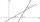# Cosine - examples

1. Isosceles triangle 8If the rate of the sides an isosceles triangle is 7:6:7, find the base angle correct to the nearest degree.
2. Trapezoid MOThe rectangular trapezoid ABCD with right angle at point B, |AC| = 12, |CD| = 8, diagonals are perpendicular to each other. Calculate the perimeter and area of ​​the trapezoid.
3. Trigonometric functionsIn right triangle is: ? Determine the value of s and c: ? ?
4. Triangle SASCalculate area and perimeter of the triangle, if the two sides are 51 cm and 110 cm long and angle them clamped is 130°.
5. Regular 5-gonCalculate area of the regular pentagon with side 7 cm.
6. ForcesIn point O acts three orthogonal forces: F1 = 20 N, F2 = 7 N and F3 = 19 N. Determine the resultant of F and the angles between F and forces F1, F2 and F3.
7. TriangleTriangle KLM is given by plane coordinates of vertices: K[-12, -11] L[-15, -18] M[-13, -12]. Calculate its area and itsinterior angles.
8. RightDetermine angles of the right triangle with the hypotenuse c and legs a, b, if: ?
9. IS triangleCalculate interior angles of the isosceles triangle with base 38 cm and legs 26 cm long.
10. ConeCalculate volume and surface area of ​​the cone with diameter of the base d = 15 cm and side of cone with the base has angle 52°.
11. PentagonCalculate the area of regular pentagon, which diagonal is u=17.
12. MastMast has 17 m long shadow on a slope rising from the mast foot in the direction of the shadow angle at angle 9.3°. Determine the height of the mast, if the sun above the horizon is at angle 44°30'.
13. RectangleCalculate the length of the side GN and diagonal QN of rectangle QGNH when given: |HN| = 25 cm and angle ∠ QGH = 28 degrees.
14. Earth parallelEarth's radius is 6374 km long. Calculate the length parallel of latitude 70°.
15. DiagonalDetermine the dimensions of the cuboid, if diagonal long 53 dm has angle with one edge 42° and with other edge 64°.
16. Rotation of the EarthCalculate the circumferential speed of the Earth's surface at a latitude of 61°​​. Consider a globe with a radius of 6378 km.
17. Rotary coneThe volume of the rotation of the cone is 472 cm3 and angle between the side of the cone and base angle is 70°. Calculate lateral surface area of this cone.
18. CuboidDetermine the dimensions of cuboid a, b, c; if diagonal d=9 dm has angle with edge a α=55° and has angle with edge b β=58°
19. Angle between linesCalculate the angle between these two lines: ? ?
20. Angle between vectorsFind the angle between the given vectors to the nearest tenth of a degree. u = (-22, 11) and v = (16, 20)

Do you have an interesting mathematical example that you can't solve it? Enter it, and we can try to solve it.

To this e-mail address, we will reply solution; solved examples are also published here. Please enter e-mail correctly and check whether you don't have a full mailbox.